### I think he did something wrong, did he?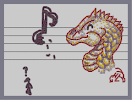Hover over the thumbnail for a full-size version.

Author Dragon_Moon author:dragon_moon bitesized n-art rated 2006-10-01 4 by 150 people. \$I think he did something wrong, did he?#Dragon_Moon#none#00000000000000000000000000000000000000000000000000000000000000000000000000000000000000000000000000000000000000000000000000000000000000000000000000000000000000000000000000000000000000000000000000000000000000000000000000000000000000000000000000000000000000000000000000000000000000000000000000000000000000000000000000000000000000000000000000000000000000000000000000000000000000000000000000000000000000000000000000000000000000000000000000000000000000000000000000000000000000000000000000000000000000000000000000000000000000000000000000000000000000000000000000000000000000000000000000000000000000000000000000000000000000000000000000000000000000000000000000000000000000000000000000000000000000000000000000000000000000000|12^516,106!12^510,110!12^505,113!12^502,117!12^500,120!12^494,126!12^490,129!12^488,132!12^483,137!12^479,142!12^475,150!12^453,177!12^547,125!12^541,128!12^533,131!12^529,133!12^524,136!12^520,141!12^514,146!12^509,151!12^504,155!12^496,162!12^492,169!12^487,174!12^481,181!12^473,188!12^470,191!12^453,187!12^453,181!12^452,177!12^446,173!12^440,174!12^435,179!12^431,183!12^430,188!12^428,194!12^423,200!12^419,205!12^475,202!12^475,197!12^470,192!12^462,191!12^457,196!12^452,201!12^451,204!12^449,208!12^444,214!12^440,218!12^436,223!12^402,209!12^398,214!12^456,239!12^471,234!12^480,231!12^488,228!12^494,223!12^497,223!12^499,224!12^504,224!12^511,225!12^518,223!12^524,218!12^525,215!12^525,213!12^404,204!12^409,198!12^413,195!12^417,191!12^430,187!12^420,184!12^426,181!12^431,177!12^436,174!12^469,154!12^467,157!12^462,162!12^458,164!12^455,166!12^454,167!12^451,170!12^400,238!12^402,241!12^407,248!12^409,253!12^413,256!12^420,259!12^427,261!12^430,265!12^435,268!12^442,269!12^453,269!12^463,263!12^470,258!12^477,254!12^484,254!12^492,254!12^500,255!12^556,190!12^549,189!12^543,190!12^535,192!12^526,196!12^521,200!12^508,254!12^504,250!12^502,247!12^502,245!12^502,245!12^592,211!12^532,106!12^537,102!12^542,95!12^546,87!12^549,80!12^556,73!12^559,67!12^565,61!12^570,55!12^575,50!12^575,50!12^575,60!12^575,70!12^575,75!12^575,82!12^575,89!12^575,98!12^575,102!12^575,107!12^578,77!12^584,74!12^590,69!12^599,64!12^603,64!12^605,81!12^611,77!12^616,73!12^624,68!12^627,74!12^627,87!12^627,98!12^627,105!12^627,109!12^632,90!12^640,86!12^648,83!12^655,84!12^654,96!12^651,105!12^648,112!12^654,99!12^667,99!12^679,97!12^677,105!12^671,114!12^665,123!12^676,114!12^687,112!12^696,112!12^699,113!12^692,127!12^688,132!12^696,130!12^705,129!12^714,129!12^718,137!12^710,144!12^705,148!12^713,146!12^727,147!12^728,149!12^719,163!12^726,156!12^522,102!12^528,99!12^531,98!12^536,97!12^538,97!10^526,172!10^526,170!10^526,166!10^527,163!10^527,162!10^530,157!10^532,155!10^534,153!10^536,151!10^539,149!10^541,149!10^544,149!10^547,150!10^549,154!10^551,156!10^553,159!10^553,160!10^554,163!10^554,166!10^554,162!10^554,160!10^554,158!10^549,153!10^546,151!10^542,151!10^537,152!10^530,158!10^528,164!10^528,167!10^527,175!10^527,176!12^563,191!12^562,192!12^567,196!12^576,198!12^583,202!12^589,205!12^593,208!12^596,212!12^599,218!12^601,221!12^602,226!12^602,230!12^603,236!12^602,243!12^598,250!12^595,254!12^591,260!12^585,266!12^579,268!12^572,271!12^563,275!12^556,276!12^549,277!12^540,275!12^531,270!12^524,266!12^517,261!12^513,258!12^510,257!12^571,197!12^420,186!12^416,190!12^416,190!12^413,195!12^410,197!12^408,199!12^406,201!12^456,164!12^459,162!12^461,161!12^464,158!12^466,157!12^468,154!12^471,151!12^474,148!12^475,147!12^477,145!12^478,143!12^480,141!12^481,139!12^483,137!12^483,136!12^485,135!12^487,132!12^488,131!12^490,128!12^491,127!12^492,125!12^493,123!12^495,121!12^498,119!12^500,116!12^503,113!12^503,113!12^506,111!12^508,109!12^510,108!12^512,106!12^515,103!12^517,102!12^519,100!12^522,98!12^525,98!12^527,98!12^530,97!12^533,95!12^536,94!12^539,94!12^540,94!12^541,94!12^541,94!12^536,96!12^531,97!12^527,97!12^526,97!12^451,169!12^451,169!12^451,169!12^531,108!12^532,107!12^534,105!12^537,102!12^538,100!12^540,98!12^542,96!12^543,95!12^544,92!12^546,89!12^548,87!12^548,85!12^550,83!12^552,79!12^553,77!12^555,75!12^558,72!12^559,70!12^560,68!12^563,66!12^565,64!12^567,62!12^568,60!12^570,57!12^571,55!12^571,54!12^573,52!12^574,51!12^575,50!12^575,104!12^575,100!12^575,97!12^575,96!12^575,93!12^575,91!12^575,89!12^575,86!12^575,82!12^575,81!12^575,78!12^575,76!12^575,74!12^575,72!12^575,70!12^575,67!12^575,65!12^575,62!12^575,60!12^575,59!12^575,57!12^575,55!12^453,181!12^451,174!12^450,172!12^448,172!12^444,172!12^441,172!12^438,176!12^437,177!12^435,180!12^432,182!12^432,185!12^429,189!12^428,191!12^426,194!12^425,196!12^423,199!12^421,200!12^420,202!12^420,202!12^436,221!12^439,216!12^442,213!12^444,211!12^446,209!12^449,207!12^451,203!12^453,200!12^455,197!12^458,195!12^459,195!12^461,193!12^463,191!12^467,187!12^469,188!12^472,193!12^474,200!12^477,187!12^477,185!12^480,182!12^482,180!12^485,178!12^486,177!12^489,175!12^490,172!12^492,170!12^494,167!12^494,166!12^498,162!12^498,161!12^500,158!12^503,154!12^505,153!12^508,150!12^509,149!12^512,146!12^514,145!12^517,142!12^519,141!12^522,139!12^524,137!12^528,134!12^530,133!12^534,130!12^540,130!12^542,129!12^547,126!12^550,124!12^553,124!12^562,123!12^559,123!12^555,123!12^553,123!12^399,218!12^399,219!12^401,222!12^406,225!12^407,227!12^410,229!12^416,232!12^420,234!12^424,235!12^429,236!12^434,236!12^439,237!12^442,238!12^447,238!12^450,238!12^454,238!12^459,238!12^460,238!12^463,238!12^467,236!12^473,235!12^477,234!12^481,233!12^488,228!12^493,225!12^494,223!12^496,222!12^500,222!12^503,223!12^506,223!12^510,224!12^513,224!12^519,224!12^520,224!12^523,222!12^524,219!12^525,216!12^483,229!12^487,229!12^488,229!12^491,226!12^492,225!12^400,226!12^400,228!12^400,231!12^400,233!12^401,235!12^402,238!12^405,242!12^405,244!12^411,248!12^413,251!12^415,254!12^417,256!12^419,256!12^421,259!12^424,260!12^426,262!12^427,263!12^430,266!12^435,270!12^439,271!12^443,271!12^449,270!12^451,269!12^457,265!12^459,264!12^464,261!12^468,259!12^474,256!12^478,255!12^482,254!12^485,254!12^491,254!12^494,254!12^496,255!12^501,256!12^505,257!12^510,258!12^512,259!12^515,260!12^515,260!12^517,260!12^517,261!12^522,264!12^526,265!12^528,266!12^531,268!12^533,270!12^535,271!12^537,272!12^539,273!12^543,273!12^544,274!12^547,275!12^550,275!12^552,275!12^555,275!12^559,275!12^563,274!12^567,273!12^571,273!12^571,273!12^575,270!12^579,269!12^580,268!12^581,267!12^584,266!12^586,263!12^588,262!12^591,260!12^595,257!12^597,256!12^600,252!12^601,249!12^603,243!12^603,241!12^603,234!12^603,232!12^603,229!12^603,224!12^600,219!12^598,217!12^595,214!12^593,212!12^590,209!12^589,207!12^585,205!12^579,201!12^578,200!12^575,198!12^572,197!12^569,195!12^566,193!12^563,193!12^560,192!12^557,191!12^554,191!12^549,192!12^545,192!12^545,192!12^541,192!12^536,192!12^534,193!12^532,193!12^530,195!12^527,196!12^525,199!12^606,226!12^609,224!12^610,222!12^615,218!12^616,217!12^620,212!12^623,209!12^624,208!12^627,205!12^630,202!12^631,200!12^633,194!12^633,190!12^633,186!12^633,180!12^632,178!12^630,176!12^628,175!12^624,172!12^622,171!12^619,171!12^615,171!12^612,170!12^610,170!12^580,77!12^582,75!12^584,74!12^585,74!12^587,72!12^589,70!12^593,67!12^597,65!12^599,63!12^601,61!12^603,60!12^604,59!12^604,59!12^604,61!12^604,66!12^604,69!12^605,73!12^605,76!12^605,79!12^605,83!12^606,91!12^606,94!12^606,96!12^606,96!12^606,99!12^606,100!12^606,101!12^606,104!12^606,105!12^606,107!12^606,109!12^606,112!12^606,114!12^606,115!12^605,88!12^605,88!12^605,86!10^246,186!10^234,186!10^222,192!10^210,198!10^204,210!10^198,222!10^198,234!10^204,246!10^210,252!10^216,258!10^228,264!10^240,264!10^252,264!10^258,258!10^264,252!10^252,246!10^246,234!10^258,228!10^246,222!10^240,210!10^246,204!10^258,198!10^270,198!10^276,198!10^276,192!10^276,180!10^276,174!10^276,156!10^276,156!10^276,150!10^276,144!10^276,144!10^276,144!10^276,156!10^276,162!10^276,168!10^276,168!10^276,174!10^276,186!10^276,192!10^276,138!10^276,132!10^276,126!10^276,114!10^276,96!10^276,78!10^258,180!10^258,174!10^258,168!10^258,162!10^258,156!10^258,144!10^258,138!10^258,132!10^258,126!10^258,126!10^258,114!10^258,108!10^258,102!10^258,96!10^258,90!10^258,84!10^258,78!10^258,72!10^258,60!10^258,54!10^264,42!10^270,42!10^276,42!10^264,252!10^262,253!10^260,255!10^259,257!10^257,259!10^255,260!10^253,261!10^251,262!10^249,263!10^246,263!10^242,263!10^239,263!10^234,261!10^232,261!10^229,261!10^227,260!10^222,257!10^220,255!10^217,253!10^215,251!10^214,250!10^212,248!10^209,246!10^206,244!10^205,243!10^202,240!10^200,237!10^199,234!10^199,230!10^199,224!10^201,220!10^201,217!10^201,213!10^204,210!10^206,208!10^209,205!10^211,203!10^211,201!10^211,199!10^214,196!10^215,195!10^220,192!10^224,190!10^227,189!10^230,187!10^234,185!10^207,200!10^205,203!10^203,205!10^203,207!10^203,211!10^203,213!10^238,183!10^243,183!10^244,183!10^247,183!10^249,183!10^253,183!10^255,183!10^256,181!10^256,178!10^256,173!10^256,170!10^256,165!10^256,162!10^256,161!10^256,157!10^256,153!10^256,150!10^256,147!10^256,144!10^256,142!10^256,141!10^256,138!10^256,135!10^256,132!10^256,128!10^256,125!10^256,123!10^256,119!10^258,113!10^258,111!10^258,107!10^258,101!10^258,96!10^259,92!10^259,88!10^259,82!10^259,79!10^259,76!10^259,70!10^259,66!10^259,64!10^261,58!10^260,55!10^260,55!10^260,49!10^261,47!10^262,47!10^265,44!10^268,44!10^270,44!10^261,251!10^259,251!10^256,250!10^253,246!10^251,243!10^248,239!10^248,235!10^249,232!10^254,228!10^255,226!10^258,226!10^254,226!10^252,226!10^248,221!10^245,219!10^244,214!10^245,209!10^246,205!10^250,201!10^255,199!10^261,197!10^264,195!10^267,195!10^271,195!10^274,195!10^275,188!10^276,184!10^276,180!10^276,177!10^276,172!10^277,166!10^277,161!10^278,155!10^278,154!10^279,148!10^279,143!10^279,137!10^279,133!10^279,126!10^279,122!10^279,119!10^279,117!10^279,114!10^279,108!10^279,105!10^279,100!10^279,98!10^279,94!10^279,91!10^279,87!10^279,85!10^279,81!10^279,78!10^279,77!10^214,242!10^217,242!10^219,237!10^221,233!10^222,230!10^222,228!10^222,224!10^222,218!10^222,215!10^224,210!10^227,208!10^233,204!10^236,203!10^239,201!10^243,199!10^245,198!10^249,197!10^251,197!10^217,198!10^218,195!10^220,194!10^224,191!10^225,191!10^261,190!10^261,188!10^259,186!10^257,186!10^252,252!10^246,252!10^240,252!10^234,252!10^228,246!10^228,252!10^222,252!10^222,246!10^234,246!10^240,246!10^246,246!10^246,258!10^234,258!10^234,258!10^240,240!10^240,234!10^240,228!10^240,222!10^240,216!10^234,216!10^234,222!10^234,228!10^234,234!10^234,240!10^228,240!10^228,228!10^228,222!10^228,234!10^228,216!10^228,210!10^234,210!10^234,204!10^240,204!10^251,254!10^244,254!10^241,254!10^233,253!10^225,248!10^220,244!10^228,245!10^237,247!10^246,245!10^242,242!10^232,243!10^226,241!10^231,234!10^238,230!10^237,243!10^227,237!10^226,228!10^229,219!10^234,211!10^236,208!10^233,224!10^242,229!10^248,229!10^250,226!10^241,223!10^239,215!10^229,259!10^280,44!10^285,46!10^291,51!10^297,53!10^299,60!10^301,69!10^303,75!10^311,78!10^318,80!10^326,81!10^333,85!10^339,95!10^341,101!10^341,107!10^341,114!10^341,125!10^338,133!10^334,141!10^330,147!10^323,152!10^316,154!10^310,154!10^304,150!10^298,143!10^300,143!10^307,143!10^314,142!10^318,137!10^322,131!10^322,123!10^322,115!10^317,108!10^310,104!10^304,102!10^297,98!10^294,95!10^290,92!10^286,87!10^283,83!10^282,79!10^340,111!10^340,106!10^340,99!10^337,92!10^335,89!10^333,86!10^326,82!10^322,80!10^319,79!10^313,79!10^309,78!10^306,74!10^304,72!10^304,67!10^302,63!10^300,59!10^298,56!10^295,53!10^290,49!10^287,47!10^285,46!10^281,46!10^280,46!10^341,119!10^341,125!10^341,127!10^340,133!10^338,136!10^334,139!10^329,144!10^325,148!10^322,152!10^319,153!10^315,153!10^308,149!10^304,145!10^301,143!10^302,143!10^311,143!10^317,139!10^319,136!10^319,129!10^320,121!10^321,115!10^319,110!10^313,106!10^309,103!10^305,100!10^299,99!10^297,98!10^293,94!10^291,91!10^288,88!10^288,84!10^326,131!10^324,123!10^323,115!10^318,108!10^316,104!10^313,100!10^309,97!10^306,95!10^303,92!10^301,90!10^298,87!10^294,83!10^292,79!10^288,75!10^284,70!10^281,68!10^277,65!10^275,64!10^273,64!10^270,64!10^294,77!10^300,77!10^304,80!10^306,83!10^308,91!10^311,96!10^314,102!10^321,107!10^330,111!10^336,116!10^328,110!10^323,104!10^320,101!10^330,109!10^323,103!10^322,100!10^321,98!10^319,97!10^318,97!10^316,96!10^315,95!10^313,149!10^318,148!10^319,145!10^323,143!10^324,138!10^326,136!10^328,134!10^328,132!10^330,130!10^331,130!10^334,127!10^334,125!10^331,124!10^330,124!10^333,125!10^333,130!10^332,138!10^319,136!10^325,128!10^326,118!10^326,133!10^317,145!10^313,148!10^329,116!10^329,116!10^334,116!10^332,114!10^267,182!10^270,182!10^269,181!10^266,183!10^267,176!10^264,169!10^265,162!10^270,168!10^271,178!10^266,180!10^266,171!10^266,167!10^270,164!10^270,156!10^270,155!10^270,151!10^265,153!10^265,158!10^267,155!10^268,148!10^268,143!10^265,138!10^265,134!10^267,146!10^267,148!10^271,145!10^271,139!10^270,134!10^270,130!10^270,128!10^264,128!10^264,129!10^262,119!10^274,126!10^274,123!10^274,122!10^274,118!10^274,115!10^274,112!10^274,107!10^274,103!10^274,99!10^274,97!10^275,94!10^276,88!10^276,84!10^276,79!10^276,75!10^272,72!10^270,71!10^268,70!10^267,73!10^265,75!10^265,75!10^257,118!10^258,115!10^257,112!10^257,109!10^257,104!10^257,99!10^257,95!10^257,90!10^257,85!10^257,81!10^258,77!10^258,72!10^258,65!10^258,62!10^260,58!10^260,53!10^261,50!10^262,44!10^266,44!10^272,44!10^276,45!10^285,47!10^289,48!10^292,50!10^296,52!10^301,56!10^265,254!10^261,255!10^256,259!10^251,260!10^247,260!10^245,260!10^241,260!10^235,261!10^231,261!10^228,261!10^222,260!10^220,257!10^217,255!10^214,252!10^211,250!10^206,248!10^202,245!10^201,242!10^200,237!10^200,233!10^199,229!10^199,226!10^199,221!10^199,217!10^204,213!10^204,210!10^204,205!10^209,200!10^212,197!10^217,194!10^222,191!10^228,188!10^232,186!10^236,184!10^240,183!10^243,183!10^268,160!10^272,160!10^275,146!10^265,141!10^265,151!10^342,110!10^343,106!10^343,101!10^342,97!10^337,92!10^335,87!10^333,85!10^327,82!10^325,81!10^331,83!10^325,150!10^321,153!10^314,155!10^309,156!10^305,151!10^304,150!10^302,148!10^284,273!10^284,274!10^285,277!10^286,279!10^287,284!10^287,286!10^287,288!10^287,289!10^283,275!10^283,273!10^283,273!10^296,240!10^297,242!10^301,243!10^303,247!10^307,249!10^307,250!10^307,250!10^304,243!10^302,242!10^301,241!10^324,334!10^326,344!10^327,346!10^327,350!10^327,345!10^326,340!10^325,337!10^325,337!10^297,328!10^295,329!10^290,333!10^286,333!10^281,331!10^281,332!10^288,334!10^293,333!9^36,168,1,0,1,6,0,0,0!9^60,168,1,0,2,6,0,0,0!9^84,168,1,0,3,6,0,0,0!9^108,168,1,0,4,6,0,0,0!9^156,168,1,0,6,6,0,0,0!9^132,168,1,0,5,6,0,0,0!9^180,168,1,0,7,6,0,0,0!9^204,168,1,0,8,6,0,0,0!9^228,168,1,0,9,6,0,0,0!9^252,168,1,0,10,6,0,0,0!9^300,168,1,0,12,6,0,0,0!9^276,168,1,0,11,6,0,0,0!9^348,168,1,0,14,6,0,0,0!9^324,168,1,0,13,6,0,0,0!9^372,168,1,0,15,6,0,0,0!9^396,168,1,0,16,6,0,0,0!9^420,168,1,0,17,6,0,0,0!9^444,168,1,0,18,6,0,0,0!9^36,216,1,0,1,8,0,0,0!9^60,216,1,0,2,8,0,0,0!9^84,216,1,0,3,8,0,0,0!9^108,216,1,0,4,8,0,0,0!9^132,216,1,0,5,8,0,0,0!9^156,216,1,0,6,8,0,0,0!9^180,216,1,0,7,8,0,0,0!9^252,216,1,0,10,8,0,0,0!9^276,216,1,0,11,8,0,0,0!9^300,216,1,0,12,8,0,0,0!9^324,216,1,0,13,8,0,0,0!9^372,216,1,0,15,8,0,0,0!9^348,216,1,0,14,8,0,0,0!9^396,216,1,0,16,8,0,0,0!9^36,264,1,0,1,10,0,0,0!9^60,264,1,0,2,10,0,0,0!9^84,264,1,0,3,10,0,0,0!9^108,264,1,0,4,10,0,0,0!9^132,264,1,0,5,10,0,0,0!9^156,264,1,0,6,10,0,0,0!9^180,264,1,0,7,10,0,0,0!9^204,264,1,0,8,10,0,0,0!9^252,264,1,0,10,10,0,0,0!9^276,264,1,0,11,10,0,0,0!9^300,264,1,0,12,10,0,0,0!9^324,264,1,0,13,10,0,0,0!9^348,264,1,0,14,10,0,0,0!9^372,264,1,0,15,10,0,0,0!9^396,264,1,0,16,10,0,0,0!9^420,264,1,0,17,10,0,0,0!9^36,312,1,0,1,12,0,0,0!9^84,312,1,0,3,12,0,0,0!9^60,312,1,0,2,12,0,0,0!9^108,312,1,0,4,12,0,0,0!9^132,312,1,0,5,12,0,0,0!9^180,312,1,0,7,12,0,0,0!9^156,312,1,0,6,12,0,0,0!9^204,312,1,0,8,12,0,0,0!9^228,312,1,0,9,12,0,0,0!9^252,312,1,0,10,12,0,0,0!9^276,312,1,0,11,12,0,0,0!9^300,312,1,0,12,12,0,0,0!9^324,312,1,0,13,12,0,0,0!9^348,312,1,0,14,12,0,0,0!9^372,312,1,0,15,12,0,0,0!9^420,312,1,0,17,12,0,0,0!9^396,312,1,0,16,12,0,0,0!9^468,312,1,0,19,12,0,0,0!9^444,312,1,0,18,12,0,0,0!9^516,312,1,0,21,12,0,0,0!9^492,312,1,0,20,12,0,0,0!10^302,93!10^300,91!10^299,88!10^299,84!10^301,80!10^301,80!10^266,65!10^266,65!10^319,121!10^319,121!10^322,119!10^304,98!10^304,98!10^298,94!10^298,88!10^298,84!10^296,82!10^292,79!10^342,101!10^342,96!10^338,91!10^334,88!10^332,86!10^327,81!10^324,81!10^319,80!10^316,79!10^313,79!10^313,79!10^305,69!10^304,65!10^302,62!10^301,60!10^300,58!10^298,57!10^274,151!10^274,157!10^272,166!10^272,172!10^272,172!10^264,170!10^264,170!10^243,205!10^238,211!10^237,216!10^237,217!10^241,211!10^237,208!10^238,205!10^240,203!10^242,202!10^246,200!10^246,200!10^253,196!10^260,196!10^264,196!10^269,196!10^271,196!10^273,196!10^273,196!10^265,173!10^262,172!10^262,152!10^262,148!10^238,256!10^234,236!10^245,237!10^250,237!10^241,237!10^239,237!10^239,225!10^239,224!10^221,245!10^260,166!10^258,169!10^258,171!10^255,170!10^255,168!10^256,168!10^259,168!10^260,168!10^266,168!10^269,168!10^272,168!10^273,167!10^274,166!10^247,265!10^245,264!10^243,263!10^240,263!10^246,263!10^249,263!10^252,261!10^253,261!10^254,261!10^254,261!10^245,216!10^244,218!10^244,222!12^635,121!12^653,126!12^663,130!12^685,148!12^697,155!12^707,164!12^701,160!12^710,170!12^715,174!12^716,177!12^720,187!12^724,193!12^727,203!12^728,209!12^729,221!12^729,236!12^727,247!12^721,259!12^717,268!12^713,276!12^708,284!12^701,294!12^696,300!12^689,307!12^683,315!12^678,320!12^610,264!12^590,302!12^568,332!12^557,354!12^570,278!12^567,289!12^556,297!12^550,303!12^539,309!12^533,313!12^521,323!12^505,345!12^501,351!12^500,356!12^498,362!12^495,368!12^496,376!12^499,398!12^498,387!12^505,408!12^517,418!12^677,361!12^680,376!9^36,360,1,0,1,14,0,0,0!9^60,360,1,0,2,14,0,0,0!9^84,360,1,0,3,14,0,0,0!9^108,360,1,0,4,14,0,0,0!9^132,360,1,0,5,14,0,0,0!9^156,360,1,0,6,14,0,0,0!9^180,360,1,0,7,14,0,0,0!9^204,360,1,0,8,14,0,0,0!9^228,360,1,0,9,14,0,0,0!9^252,360,1,0,10,14,0,0,0!9^276,360,1,0,11,14,0,0,0!9^300,360,1,0,12,14,0,0,0!9^324,360,1,0,13,14,0,0,0!9^348,360,1,0,14,14,0,0,0!9^396,360,1,0,16,14,0,0,0!9^372,360,1,0,15,14,0,0,0!9^420,360,1,0,17,14,0,0,0!9^444,360,1,0,18,14,0,0,0!9^468,360,1,0,19,14,0,0,0!9^492,360,1,0,20,14,0,0,0!9^756,168,1,0,31,6,0,0,0!9^756,216,1,0,31,8,0,0,0!9^756,264,1,0,31,10,0,0,0!9^732,264,1,0,30,10,0,0,0!9^756,312,1,0,31,12,0,0,0!9^732,312,1,0,30,12,0,0,0!9^708,312,1,0,29,12,0,0,0!9^756,360,1,0,31,14,0,0,0!9^732,360,1,0,30,14,0,0,0!9^708,360,1,0,29,14,0,0,0!9^684,360,1,0,28,14,0,0,0!10^167,470!10^167,471!10^171,473!10^173,474!10^174,476!10^175,477!10^177,478!10^176,479!10^171,480!10^168,481!10^164,483!10^161,485!10^157,486!10^155,487!10^152,488!10^150,489!10^153,487!10^158,484!10^161,482!10^164,480!10^166,478!10^166,477!10^167,476!10^164,476!10^162,477!10^163,474!10^162,475!10^156,481!10^155,482!10^164,482!10^168,479!4^156,450,1!10^172,489!10^173,491!10^173,493!10^173,495!10^173,497!10^173,502!10^172,505!10^172,507!10^172,511!10^172,515!10^174,522!10^173,523!10^174,526!10^177,528!10^177,531!10^178,534!10^179,536!10^179,540!10^179,542!10^179,545!10^179,547!10^179,551!10^179,554!10^179,557!10^179,557!10^179,560!10^179,563!10^179,565!10^178,565!10^176,563!10^172,561!10^172,560!10^176,558!10^176,555!10^176,558!10^174,558!10^175,552!10^175,549!10^170,529!10^170,534!10^166,540!10^166,543!10^166,547!10^164,551!10^163,555!10^162,558!10^159,555!10^159,551!10^160,547!10^161,545!10^179,504!10^181,506!10^182,508!10^184,510!10^185,514!10^187,519!10^188,522!10^190,525!10^190,527!10^166,503!10^164,508!10^165,508!10^162,513!10^160,517!10^160,518!10^160,520!10^160,522!10^160,525!10^172,530!10^172,529!10^172,527!10^172,524!10^172,521!10^172,516!10^172,511!10^172,507!10^172,502!10^172,497!10^172,488!10^185,565!10^185,565!10^185,561!10^183,559!10^182,558!10^180,556!10^166,537!10^143,412!10^144,411!10^146,409!10^150,406!10^153,406!10^155,406!10^158,407!10^160,410!10^161,412!10^160,415!10^157,417!10^156,418!10^156,420!10^156,425!10^156,428!10^156,429!10^156,430!10^156,433!10^156,433!10^156,429!10^156,426!10^142,411!10^143,411!10^145,411!10^145,410!10^147,409!10^150,407!10^151,407!10^154,407!10^156,408!10^143,409!10^144,409!10^146,407!10^149,406!10^150,405!10^153,405!10^156,405!10^157,406!10^157,407!10^158,408!10^160,408!10^161,410!10^161,411!10^161,414!10^162,416!10^162,417!10^160,419!10^159,420!10^157,421!10^156,423!10^155,424!10^155,426!10^155,427!10^155,430!10^155,431!10^155,432!10^155,433!10^155,433!10^156,436!10^156,436!10^156,437!10^156,437!10^156,453!10^156,454!10^155,453!10^155,452!10^155,451!10^155,451!10^155,451!12^680,315!12^689,307!12^693,304!12^702,293!12^703,292!12^704,290!12^705,289!12^706,288!12^707,285!12^708,284!12^709,282!12^710,280!12^712,280!12^712,278!12^712,276!12^713,273!12^715,271!12^716,269!12^716,269!12^718,266!12^720,261!12^721,261!12^722,259!12^723,257!12^724,254!12^725,251!12^725,250!12^726,245!12^726,242!12^727,241!12^728,240!12^729,238!12^729,235!12^729,232!12^729,227!12^730,223!12^730,221!12^730,218!12^730,211!12^730,207!12^730,203!12^726,199!12^725,197!12^724,194!12^723,193!12^722,189!12^721,187!12^720,183!12^718,180!12^716,178!12^714,176!12^711,173!12^708,169!12^705,165!12^702,162!12^701,161!12^698,158!12^696,156!12^694,156!12^692,154!12^690,152!12^688,150!12^686,148!12^684,146!12^681,143!12^679,141!12^677,140!12^676,139!12^674,138!12^673,137!12^671,136!12^669,135!12^667,133!12^665,131!12^662,130!12^661,129!12^660,128!12^657,127!12^655,126!12^651,124!12^647,124!12^645,124!12^643,122!12^640,121!12^637,121!12^636,121!12^729,203!12^728,201!12^727,197!12^723,193!12^723,192!12^722,191!12^721,188!12^720,187!12^720,183!12^731,214!12^731,216!12^731,218!12^628,108!12^627,107!12^626,104!12^626,102!12^626,101!12^626,98!12^626,95!12^626,92!12^626,90!12^626,89!12^626,86!12^626,83!12^626,81!12^626,80!12^626,77!12^626,75!12^626,72!12^626,70!12^622,72!12^618,73!12^616,75!12^613,77!12^611,78!12^611,78!12^617,74!12^620,72!12^621,71!12^621,71!12^645,116!12^645,115!12^645,114!12^647,111!12^648,108!12^650,104!12^650,100!12^650,100!12^651,97!12^651,94!12^652,92!12^653,90!12^655,88!12^656,87!12^657,84!12^657,83!12^658,82!12^631,91!12^634,89!12^637,87!12^647,83!12^648,83!12^650,83!12^645,85!12^658,99!12^658,99!12^662,99!12^664,99!12^666,99!12^668,99!12^672,99!12^676,99!12^678,99!12^678,100!12^676,103!12^674,107!12^674,109!12^672,112!12^670,115!12^667,119!12^664,123!12^679,114!12^684,114!12^685,114!12^688,114!12^692,114!12^696,114!12^699,114!12^699,115!12^696,121!12^694,123!12^691,127!12^687,132!12^684,135!12^682,139!12^695,129!12^698,129!12^701,129!12^704,129!12^708,129!12^711,129!12^715,129!12^718,129!12^722,129!12^723,129!12^720,135!12^716,139!12^714,141!12^711,144!12^708,147!12^706,150!12^705,152!12^705,152!12^701,153!12^717,169!12^718,167!12^723,163!12^725,161!12^726,158!12^727,156!12^729,153!12^729,151!12^731,147!12^732,146!12^727,147!12^724,147!12^719,147!12^717,147!12^714,147!12^730,163!12^732,162!12^736,162!12^737,164!12^735,169!12^734,171!12^731,174!12^728,178!12^726,181!12^725,183!12^732,177!12^736,178!12^738,179!12^737,182!12^735,184!12^734,186!12^732,190!12^732,191!12^731,196!12^568,284!12^568,285!12^567,286!12^564,289!12^563,291!12^563,293!12^566,295!12^569,299!12^571,299!12^573,299!12^578,300!12^582,300!12^584,300!12^587,300!12^593,300!12^597,299!12^601,297!12^603,296!12^609,293!12^611,290!12^616,287!12^619,285!12^620,283!12^621,282!12^602,295!12^603,295!12^604,294!12^586,267!12^589,267!12^591,267!12^597,267!12^599,267!12^601,266!12^606,265!12^610,264!12^615,260!12^617,258!12^621,256!12^624,253!12^624,250!12^624,245!12^624,242!12^624,240!12^622,237!12^621,237!12^624,278!12^624,277!12^624,276!12^623,272!12^622,270!12^622,268!12^621,267!12^622,236!12^619,235!12^617,234!12^614,233!12^612,233!12^610,233!12^619,264!12^619,264!12^559,294!12^557,297!12^553,299!12^550,301!12^548,302!12^547,303!12^544,304!12^543,305!12^541,307!12^538,308!12^537,309!12^536,313!12^536,315!12^538,319!12^542,321!12^547,325!12^549,326!12^553,327!12^555,328!12^556,328!12^559,329!12^562,329!12^564,330!12^569,331!12^572,331!12^576,331!12^579,331!12^582,331!12^587,330!12^592,326!12^612,313!12^616,309!12^617,307!12^617,303!12^617,300!12^617,299!12^617,298!12^617,295!12^617,295!12^616,293!12^616,293!12^532,317!12^528,318!12^525,320!12^522,321!12^519,322!12^515,323!12^513,325!12^510,330!12^507,333!12^505,336!12^503,341!12^503,344!12^505,348!12^512,350!12^516,354!12^520,355!12^524,355!12^531,355!12^535,355!12^538,355!12^542,355!12^548,355!12^552,355!12^555,355!12^558,355!12^564,355!12^568,354!12^572,352!12^576,350!12^580,348!12^583,346!12^586,343!12^588,341!12^588,337!12^588,336!12^588,335!12^502,349!12^501,352!12^500,357!12^498,360!12^496,362!12^496,365!12^496,370!12^497,373!12^497,378!12^497,380!12^499,385!12^502,387!12^506,390!12^509,391!12^512,391!12^516,392!12^519,392!12^522,393!12^525,393!12^527,393!12^531,393!12^534,393!12^537,393!12^542,391!12^545,390!12^549,389!12^551,389!12^554,388!12^558,387!12^559,386!12^563,383!12^570,380!12^572,379!12^577,375!12^580,371!12^581,370!12^582,368!12^582,364!12^582,360!12^582,357!12^582,355!12^580,353!12^578,352!12^565,384!12^501,392!12^501,393!12^500,395!12^500,398!12^502,401!12^504,405!12^507,409!12^509,412!12^511,415!12^514,416!12^518,419!12^520,420!12^522,420!12^526,422!12^529,424!12^532,427!12^536,428!12^541,428!12^543,428!12^547,428!12^551,427!12^556,424!12^561,421!12^567,417!12^572,415!12^577,412!12^580,411!12^583,408!12^584,406!12^588,401!12^589,396!12^589,392!12^589,388!12^587,386!12^585,383!12^582,381!12^579,380!12^576,380!12^591,329!12^594,327!12^596,325!12^598,323!12^600,322!12^603,320!12^605,319!12^606,317!12^609,315!12^611,312!12^613,311!12^616,309!12^699,300!12^695,300!12^692,303!12^689,307!12^688,309!12^685,312!12^684,312!12^680,316!12^679,318!12^678,319!12^678,322!12^675,327!12^673,331!12^673,334!12^673,340!12^673,342!12^675,347!12^675,353!12^676,356!12^677,362!12^678,364!12^679,367!12^682,374!12^683,376!12^684,378!12^699,297!12^699,297!12^676,326!12^676,326!12^682,375!12^682,375!12^680,372!12^679,371!12^679,369!12^679,367!12^679,364!12^677,361!12^675,358!12^674,354!12^674,351!12^674,348!12^674,344!12^674,338!9^732,216,1,0,30,8,0,0,0!9^732,168,1,0,30,6,0,0,0!12^720,167!12^734,168!12^730,217!9^684,312,1,0,28,12,0,0,0!12^688,312!12^687,312!12^686,312!12^685,312!12^685,312!12^683,312!12^683,312!12^689,309!3^648,195!3^651,200!3^658,203!3^665,204!3^670,203!3^674,195!3^674,190!3^674,185!3^669,182!3^663,176!3^661,174!3^684,195!3^687,195!3^692,195!3^697,194!3^701,189!3^701,183!3^700,178!3^695,173!3^691,170!3^706,204!3^707,207!3^708,212!3^711,214!3^716,214!3^722,214!3^723,213!3^701,197!3^674,215!3^676,223!3^678,230!3^682,233!3^687,233!3^692,233!3^696,232!3^700,227!3^706,221!3^708,218!3^676,221!3^672,215!3^670,210!3^701,239!3^701,245!3^699,253!3^699,257!3^703,260!3^707,260!3^714,260!3^716,258!3^646,194!3^644,192!3^642,189!3^659,217!3^658,222!3^653,224!3^648,226!3^644,226!3^641,224!3^638,221!3^635,218!3^634,215!3^631,213!3^648,233!3^648,239!3^648,246!3^647,250!3^645,251!3^641,253!3^637,253!3^633,251!3^632,249!3^679,239!3^677,244!3^673,250!3^668,254!3^666,256!3^663,256!3^658,252!3^654,249!3^669,263!3^667,267!3^666,273!3^666,277!3^668,280!3^673,280!3^678,279!3^684,274!3^687,272!3^694,268!3^698,264!3^667,287!3^667,292!3^667,299!3^667,306!3^670,309!3^675,309!3^679,309!3^681,309!3^671,287!3^630,287!3^635,284!3^639,281!3^641,279!3^643,277!3^644,274!3^648,269!3^650,266!3^650,262!3^650,262!3^626,282!3^634,293!3^634,299!3^634,303!3^634,305!3^635,308!3^639,309!3^643,309!3^646,309!3^649,309!3^653,309!3^656,306!3^658,304!3^637,322!3^639,329!3^640,334!3^642,340!3^642,345!3^644,348!3^647,353!3^651,354!3^654,354!3^658,354!3^661,352!3^665,350!3^666,349!3^639,319!3^639,325!3^639,333!3^638,316!3^637,341!3^634,342!3^630,343!3^623,344!3^619,342!3^616,340!3^613,336!3^611,333!3^610,330!3^610,328!3^611,325!3^617,347!3^617,353!3^616,358!3^614,362!3^611,363!3^607,366!3^605,368!3^602,369!3^597,368!3^594,366!3^592,364!3^591,362!3^617,375!3^621,381!3^624,385!3^627,389!3^630,392!3^633,393!3^638,393!3^643,393!3^646,391!3^650,388!3^654,382!3^654,377!3^656,372!3^656,365!3^656,363!3^612,373!3^612,372!3^632,400!3^630,404!3^627,408!3^620,409!3^612,409!3^606,408!3^601,404!3^597,401!3^596,400!3^627,420!3^624,436!3^605,436!3^594,426!3^612,436!3^626,429!3^639,415!3^655,423!3^665,416!3^670,404!3^662,395!3^649,419!3^684,399!3^685,388!3^650,145!3^657,155!3^652,168!3^632,165!3^642,166!3^669,165!3^678,160!3^686,153!3^686,164!3^640,130!3^657,133!3^507,247!3^516,252!3^525,257!3^535,260!3^543,264!3^554,264!3^566,263!3^576,258!3^585,252!3^592,245!3^556,257!3^536,253!3^518,240!3^572,248!3^531,237!3^532,223!3^547,241!3^593,230!3^585,217!3^570,206!3^556,200!3^538,201!3^489,247!3^476,248!3^464,253!3^454,262!3^444,263!3^435,255!3^425,249!3^414,241!3^407,210!3^408,219!3^421,227!3^437,229!3^450,229!3^460,225!3^473,222!3^486,219!3^501,216!3^514,218!3^506,203!3^490,209!3^421,214!3^471,180!3^480,171!3^488,159!3^498,150!3^506,143!3^513,137!3^524,130!3^534,122!3^554,117!3^545,116!3^525,119!3^507,128!3^477,161!3^462,173!3^461,187!3^443,185!3^444,182!3^434,195!3^427,204!3^448,188!3^444,181!3^436,187!3^432,200!3^478,192!3^488,185!3^494,179!3^495,172!3^502,166!3^507,160!3^516,152!3^529,141!3^538,136!3^549,131!3^558,130!3^561,141!3^575,133!3^569,123!3^585,126!3^602,126!3^511,175!3^495,192!3^565,185!3^575,190!3^585,195!3^596,204!3^545,185!3^569,103!3^569,98!3^569,93!3^569,86!3^569,81!3^570,74!3^571,70!3^571,66!3^600,107!3^599,100!3^599,94!3^599,88!3^599,83!3^599,75!3^599,75!3^623,103!3^623,94!3^623,88!3^623,82!3^560,104!3^556,104!3^551,98!3^590,104!3^584,99!3^582,97!3^544,107!3^566,114!3^587,113!3^581,88!3^613,111!3^623,120!3^638,113!3^641,102!3^657,119!3^667,110!3^647,93!3^675,129!3^680,134!3^687,124!3^693,146!3^705,144!3^711,140!3^712,162!3^718,157!3^724,174!3^730,184!3^610,214!3^614,209!3^617,207!3^622,202!3^625,199!3^626,189!3^626,179!3^614,177!3^607,201!3^613,192!3^601,168!10^152,485!10^153,484!10^154,483!10^157,480!10^157,480!10^159,478!10^159,476!10^160,475!10^162,473!10^163,472!10^164,472!10^165,471!10^166,470!10^169,470!10^170,470!10^172,472!10^172,473!10^174,475!10^176,476!10^176,476!10^149,489!10^191,526!10^190,523!10^189,521!10^189,519!10^188,518!10^186,514!10^185,513!10^184,512!10^183,509!10^182,507!10^180,505!10^176,502!10^175,502!10^159,522!10^159,520!10^162,516!10^163,514!10^164,511!10^166,509!10^168,505!10^177,550!10^177,543!10^177,539!10^182,556!10^181,551!10^181,566!0^509,358!0^504,369!0^509,378!0^525,385!0^541,384!0^524,374!0^518,360!0^551,380!0^564,374!0^575,364!0^536,374!0^512,384!0^530,327!0^521,333!0^519,342!0^531,343!0^545,348!0^559,348!0^571,348!0^563,303!0^550,309!0^553,322!0^561,315!0^571,324!0^583,323!0^596,314!0^580,277!0^577,289!0^509,341!0^522,350!0^588,291!0^599,291!0^585,283!0^604,288!0^601,259!0^610,254!0^615,249!0^612,243!0^611,269!0^593,274!0^517,404!0^530,411!0^539,423!0^556,416!0^567,409!0^578,401!0^545,414!0^528,402!0^544,408!0^578,391!0^508,398!0^521,413!0^556,410!0^607,305!0^536,344!0^533,344!0^530,342!0^526,341!0^521,338!0^519,336!0^519,336!0^523,337!0^534,341!0^528,381!0^526,380!0^523,378!0^519,377!0^515,375!0^510,373!0^509,371!0^541,415!0^540,415!0^536,415!0^533,415!0^531,413!0^527,411!0^524,410!3^521,338!3^524,341!3^532,344!3^529,380!3^522,380!3^515,380!3^513,378!3^510,373!3^548,417!3^542,418!3^537,418!3^529,415!3^522,410!3^580,336!3^571,336!3^561,336!3^554,332!3^548,328!3^539,323!3^573,358!3^562,358!3^551,359!3^539,359!3^525,359!3^513,354!3^576,383!3^566,388!3^553,391!3^541,395!3^526,395!3^509,396!3^516,395!3^605,298!3^596,301!3^584,302!3^573,300!3^565,298!3^613,267!3^602,268!3^595,271!3^584,272!3^610,243!3^610,244!3^605,249!3^603,252!3^600,256!3^596,260!3^593,262!3^615,243!3^591,277!3^587,280!3^583,283!3^581,288!3^581,292!3^581,293!3^575,290!3^575,285!3^579,279!3^579,277!3^583,275!3^563,305!3^560,305!3^553,307!3^547,312!3^545,314!3^559,300!3^581,320!3^572,320!3^567,374!3^562,377!3^575,402!3^570,405!3^610,282!0^645,382!0^632,379!0^626,369!0^628,364!0^637,371!0^628,358!0^660,338!0^652,334!0^650,322!0^661,334!0^604,356!0^594,354!0^591,344!0^598,350!0^627,331!0^619,321!0^621,398!0^608,392!0^598,386!0^593,378!0^690,299!0^683,299!0^680,294!0^655,295!0^645,296!0^647,287!0^633,273!0^630,266!0^636,236!0^628,232!0^622,229!0^650,215!0^639,209!0^665,194!0^649,184!0^691,187!0^680,178!0^671,241!0^665,241!0^658,236!0^698,218!0^691,222!0^684,218!0^684,213!0^714,244!0^714,239!0^717,205!0^712,200!0^711,197!0^711,252!0^717,252!0^685,286!0^693,295!0^706,268!0^696,275!0^658,342!0^667,329!0^672,318!0^649,320!0^649,331!0^651,340!0^655,344!0^666,386!0^674,394!0^678,381!0^665,378!0^669,357!0^593,416!0^610,426!0^619,422!0^643,405!0^653,409!0^660,406!0^644,381!0^640,381!0^633,375!0^624,367!0^625,357!0^649,376!0^649,368!0^649,362!0^635,352!0^638,382!0^631,375!0^621,401!0^614,398!0^607,395!0^602,392!0^598,389!0^593,381!0^590,374!0^589,371!0^620,393!0^615,386!0^586,378!0^600,356!0^593,354!0^592,353!0^609,353!0^609,347!0^607,344!0^630,332!0^595,338!0^590,351!0^623,332!0^622,332!0^621,326!0^618,321!0^617,318!0^630,328!0^620,311!0^657,295!0^653,297!0^648,298!0^643,297!0^643,291!0^645,286!0^652,277!0^654,269!0^648,299!0^638,270!0^633,271!0^629,271!0^626,266!0^626,264!0^687,262!0^684,268!0^679,270!0^677,265!0^679,261!0^680,258!0^681,260!0^684,263!0^684,254!0^665,242!0^662,241!0^662,236!0^661,232!0^667,240!0^638,239!0^634,235!0^630,231!0^626,229!0^621,226!0^621,224!0^625,228!0^650,215!0^644,213!0^639,208!0^638,208!0^664,195!0^659,191!0^656,189!0^652,186!0^650,184!0^667,192!0^691,184!0^687,184!0^683,182!0^680,180!0^677,177!0^698,218!0^696,218!0^691,215!0^687,212!0^684,208!0^682,205!0^702,216!0^716,201!0^710,196!0^713,249!0^712,245!0^708,239!0^707,236!0^723,235!0^712,230!0^719,223!0^679,292!0^679,297!0^682,299!0^692,282!0^675,302!0^675,296!3^670,377!3^660,340!3^667,321!3^688,297!3^701,279!3^715,250!3^715,228!3^715,193!3^668,356!3^665,335!3^675,310!3^695,288!3^708,266!3^719,239!3^720,223!3^719,206!3^709,181!3^671,366!0^585,247!0^574,257!0^565,261!0^555,261!0^538,259!0^526,252!0^503,247!0^516,253!0^556,272!0^598,232!0^586,217!0^574,206!0^564,199!0^551,199!0^534,199!0^542,248!0^536,234!0^536,233!0^522,244!0^499,248!0^461,256!0^443,260!0^435,257!0^412,238!0^403,231!0^416,239!0^441,251!0^571,242!0^542,233!0^558,241!0^537,220!0^580,236!0^486,246!0^466,253!0^455,252!0^509,217!0^482,223!0^460,227!0^433,228!0^423,221!0^411,212!0^412,207!3^438,196!3^448,195!3^439,208!0^464,175!0^480,176!0^482,161!0^499,147!0^516,135!0^478,149!0^492,136!0^519,106!0^528,126!0^544,115!0^552,101!0^564,113!0^567,94!0^567,77!0^567,67!0^554,134!0^530,145!0^501,171!0^570,191!0^607,209!0^627,190!0^616,174!0^616,197!0^570,180!0^567,162!0^594,185!0^643,166!0^655,170!0^593,119!0^602,105!0^596,82!0^621,108!0^620,92!0^633,111!0^640,101!0^647,117!0^661,120!0^680,135!0^683,128!0^699,145!0^715,158!0^725,174!0^698,165!0^668,148!0^644,134!0^631,125!0^615,123!0^622,134!0^640,145!0^664,160!0^628,153!0^607,139!0^585,171!0^609,161!0^582,150!0^565,136!0^579,128!12^695,438!12^692,440!12^691,442!12^689,445!12^687,447!12^686,449!12^683,452!12^682,455!12^680,458!12^677,461!12^676,462!12^708,442!12^707,443!12^703,447!12^702,448!12^699,452!12^698,454!12^696,457!12^694,459!12^693,460!12^693,460!12^720,445!12^719,449!12^716,452!12^714,455!12^712,459!12^710,460!12^709,461!12^706,465!12^703,468!12^702,470!12^699,472!12^698,475!12^696,476!12^694,479!12^693,480!12^692,482!12^690,485!12^690,485!12^692,486!12^695,486!12^697,486!12^700,485!12^703,485!12^706,485!12^709,485!12^711,485!12^713,485!12^691,466!12^696,454!12^691,454!12^687,454!12^683,454!12^679,454!12^676,454!12^672,454!12^670,455!12^667,458!12^665,461!12^661,466!12^660,468!12^660,469!12^660,474!12^661,480!12^664,483!12^669,488!12^671,488!12^674,489!12^673,477!12^673,481!12^673,482!12^675,484!12^671,490!12^666,490!12^662,490!12^660,492!12^717,460!12^721,461!12^724,462!12^729,465!12^731,468!12^735,473!12^737,477!12^737,478!12^738,484!12^738,487!12^738,491!12^743,491!12^746,490!12^750,485!12^749,481!12^749,479!12^749,476!12^748,472!12^745,469!12^743,468!12^737,467!12^739,465!12^739,457!12^739,446!12^736,436!12^733,432!12^730,428!12^728,424!12^723,418!12^720,416!12^717,413!12^715,412!12^710,409!12^706,408!12^742,460!12^742,454!12^742,450!12^740,446!12^736,441!12^735,439!12^733,437!12^729,434!12^726,432!12^723,432!12^721,431!12^715,429!12^710,429!12^706,429!12^702,429!12^705,410!12^701,410!12^699,410!12^696,410!12^695,410!12^726,421# My new n art. isnt he cute? Please dont see it as a comeback or something like that. School is getting harder and harder and i dont have that much time. But sometimes ill submit some maps of course ;)

## Other maps by this author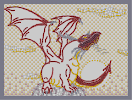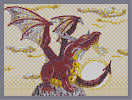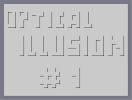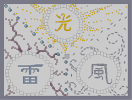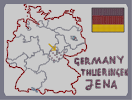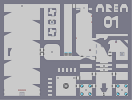Afternoondragon Step03 Afternoondragon Step04 illusion #1 Ming Lei Feng/Light Thunder Wind Here I am DARK STORM -- Area 01

### ...funny...just...hilarious...

im just gonna say it...to me, its a dissapointment compared to some of your other arts...

dont get me wrong, its very good...but its just not THAT great...i dont actually have hardly anything to say against it, which is a tribute to your skill, but its just too simplistic, nothing compared to your previous complexity...

oh, one thing of critisism, the try at a 3d looking head(can see front and side) didnt work very well for me, there seems to be a difference between the jaw and rest of head(no 3d, attempt at 3d)

alright, this is rather long, and im sure most ppl will think im an asshole for critisizing and that you are still godlike regardless of what i say, so i take my leave

### love it

lol i like the N guy he's just like HUH?

### Well,

the dragon's scales are incredibly realistic, and the note is too. 4.5

### WOW!

Wow is all I can say!

### WOW

every piece of art i've seen here is spectacular! NICE ONE DRAGON_MOON! i rate it a >5/5<

Like dragon ^.^

### what the

when the map is loaded why is the lasers trying to shoot the ninja art

### sweet!!!

I got the 100th vote!!!!

And just for that it's a 5

### wait

is that the body of the dragon at the bottom

### @neme

http://metanet.2.forumer.com/index.php?showtopic=13334
he's gone.

### um

bobo?

you feeling alright?

### pretty good

but not as awesome as some of your other ones.
4.5/5

### freakin awesome

5aved, but what's the point?

### Hello

This art is a bit messy and unappealing to me. Sorry about that. It simply has an incomplete feel to it. 4.5.

### thumbs down from me

it sucks compared to your other ones. zero out of five. and check out this website: www.penisbot.com

period

### @alex

if a map has been rated five 5's, even a 4.5 will lower it.

5/5

that rocks...
:)
5/5

### well

you mean the ninja?
Cuz i think that improves the art. I thought you were talking about the funny red thing below the dragon.

### ARG

I dont think there is ever going to be a map with 5/5. Do many stupid snipers.

Coz this is very very nice. :) 5/5 from me

### Actually

if you weren't being sarcastic, it is the left.

### 99turtle99

there was nothing on the bottom left. Were you perhaps referring to the bottom _right_ ?
=P

### awesome

that has got to be the most amazing n art ive ever seen

### lol

yea...its a confused ninja...

and im glad ppl actually understand me this time around(usually i get a bunch of hate messages)...

but, i have to dissapoint you for now dragon(this goes to turtle too)...i really jsut dont have any time now to finish it...and i honestly cant imagine when i am going to get time...and i also have a bad feeling i need to remake almost half of it...i just cant waste time like that now...ive been saying similar things for a while...but its true...realistically...it might not be done till christmas, but i swear ill try to work it in well before then, but at this rate, it looks unlikely...sry guys...i wish there was more time in the day...

### ...turtle, its N, that ninja and he's confused why a happy dragon

is eating a note...

### that thing on the bottom left really messes it up for me

:(

I love it otherwise

dont rate it 4.5/5 or less, because even if you didnt think it was DM's best work, it still kills everyone elses (no offence to the public)

### 4.5

very clean and well rounded. Straight to the point and it still looks nice in full screen.
But it doesnt maximize its potential and is a little simple compared to your others

### nah

i'll listen to you, nemetacyst.

### lol

man, thats the reason why i like u so much :D
I think there is nothing wrong on critisising. But please please please can u finish ur three-dragon-picture? Im w8ting so long :D

@lord_day
this is my personal sign.

### 5aved.

If someone snipes this, I will personally rip out their...uh...never mind.

5aved.

### freaking

amazing.
as always.
you're awesome.
5/5, and may it stay that way forever.

Look sweet =)

### Sorry to double comment, but...

bitesized!!!!!!!!!!!!

### holy crap

I know what its like with school and everything, but you deserve a break, the map on the other hand is amazing and kinda funny, N didnt apear very well tho 4.5/5

5/5

### wow...

I think it's very very good! 5/5